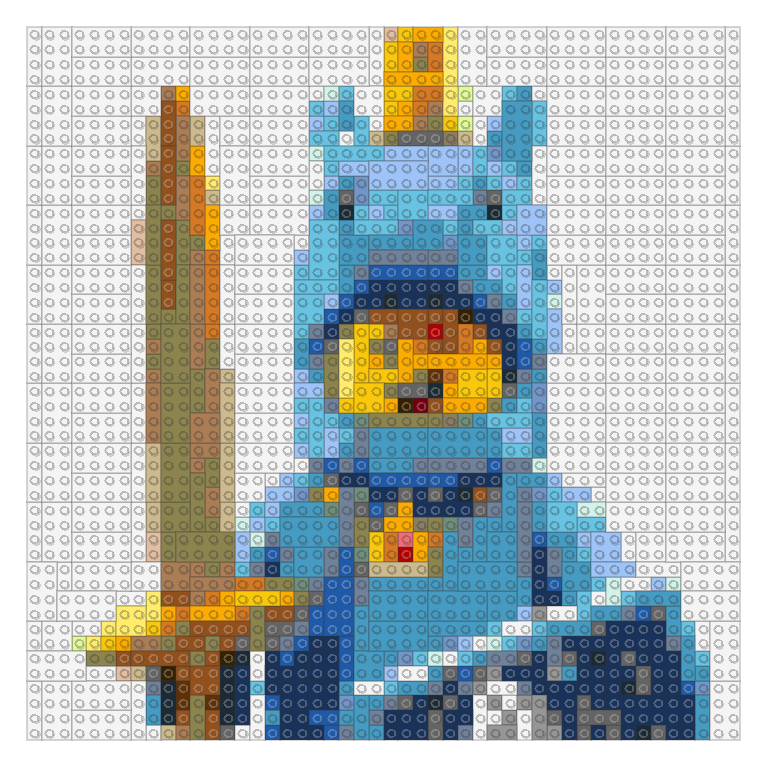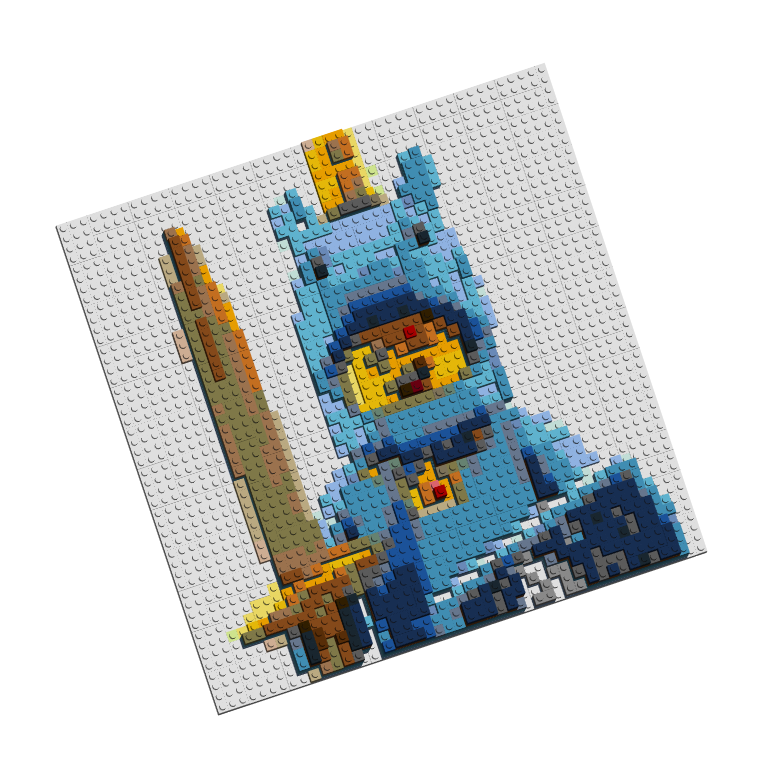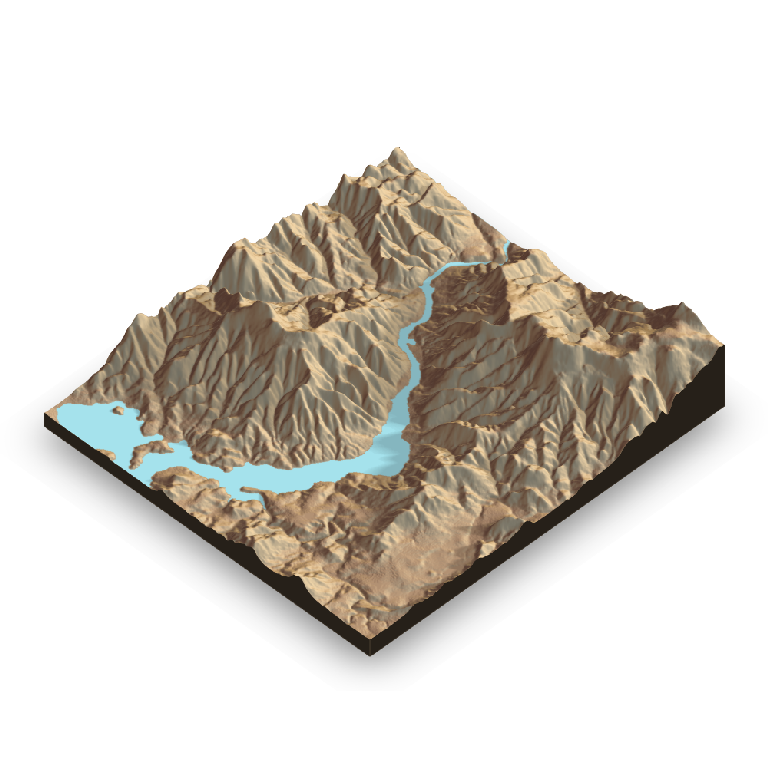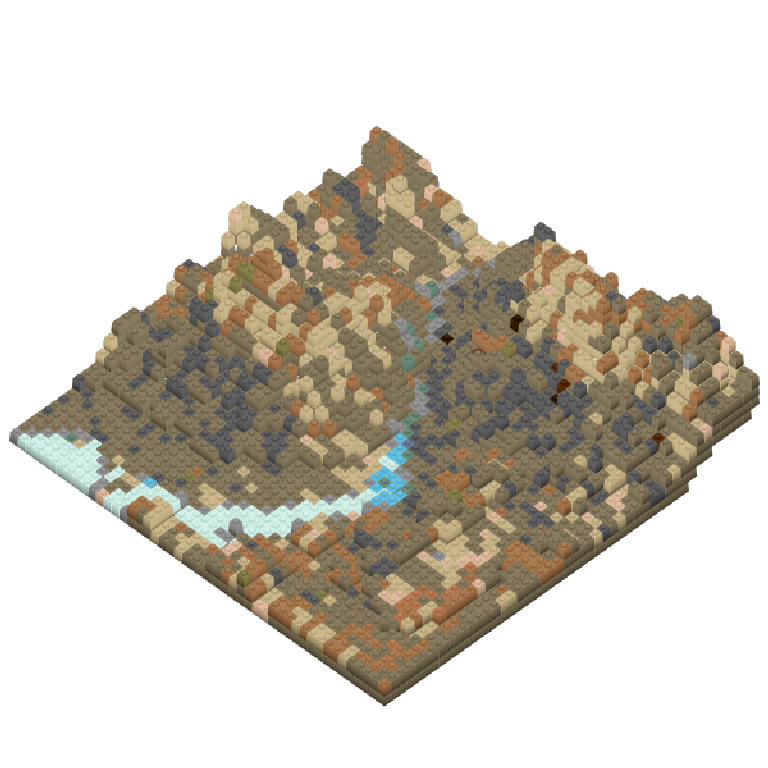## Getting started

The bricks_from_* series of functions creates 3D models of LEGO bricks from a variety of input formats.

## 3D mosaics

Begin with a brickr mosaic from an image. Rather than graphically rendering the mosaic using build_mosaic(), use bricks_from_mosaic(). This function takes two other inputs:

• mosaic_height is the number of bricks stacked at the mosaic’s highest point. The default is 6.
• highest_el specifies if ‘light’ or ‘dark’ color bricks should be the tallest in the model. The default is ‘light’.
demo_img = tempfile()

image_to_mosaic()

mosaic %>% build_mosaic()mosaic %>%
bricks_from_mosaic(highest_el = "dark") %>%
build_bricks()

#From dput(round(rgl::par3d("userMatrix"),1)) after manual rotation
custom_rotation <- structure(c(0.9, 0.3, -0.3, 0, -0.3, 0.9, -0.3,
0, 0.2, 0.4, 0.9, 0, 0, 0, 0, 1), .Dim = c(4L, 4L))

rgl::par3d(userMatrix = rgl::rotate3d(custom_rotation, 0, 0, pi/4 ,1))rayshader by Tyler Morgan-Wall is an open source package for producing 2D and 3D data visualizations in R. rayshader uses elevation data in a base R matrix and a combination of raytracing, spherical texture mapping, overlays, and ambient occlusion to generate beautiful topographic 2D and 3D maps. (Note: text lifted straight from rayshader.com.)

3D models in brickr are rendered using the functions in rayshader. Using bricks_from_rayshader(), you can convert rayshader map output into a brickr model. This function takes three inputs:

• hillshade is topographic image matrix with an RGB channel (much like the mosaics).
• heightmap is a two-dimensional matrix specifiying the height of the image at each location.
• max_height is the number of bricks stacked at the mosaic’s highest point. The default is 12.
• img_size is the number of bricks on each side of the model. The default is 48.
library(rayshader)

#Here, I load a map with the raster package.

#And convert it to a matrix:
elmat = matrix(raster::extract(localtif, raster::extent(localtif), buffer = 1000),
nrow = ncol(localtif), ncol = nrow(localtif))

plot_3d(elmat, zscale = 10, fov = 0, theta = 135, zoom = 0.75, phi = 45, windowsize = c(1000, 800))

rayshader::render_snapshot(clear = TRUE)#Plot as bricks
rayshader::render_snapshot(clear = TRUE)This example is rendered using build_bricks_rayshader(), which a bit faster for very large sets.